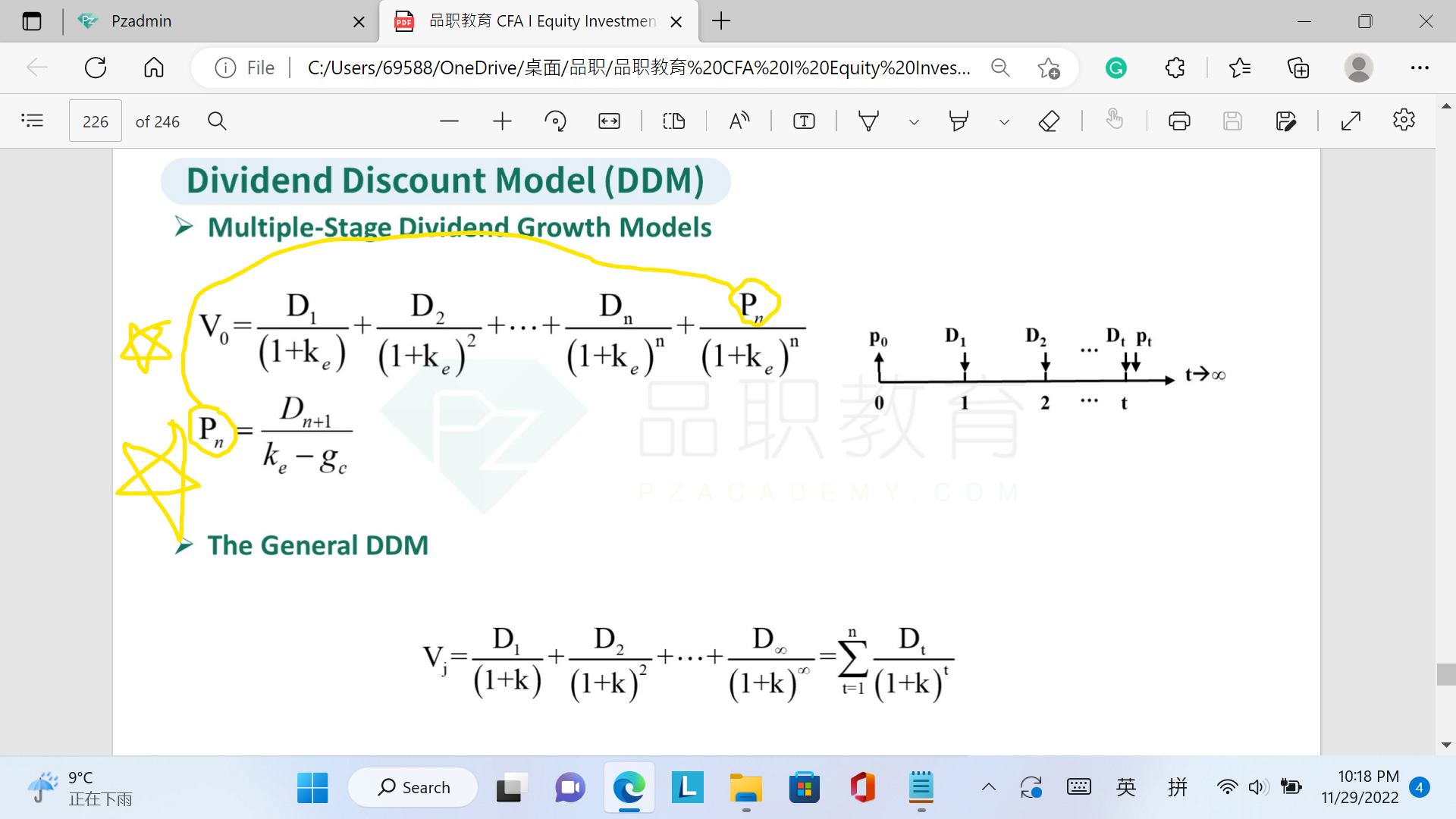# 根据讲义 DDM的公式不是不需要看P吗

NO.PZ2015122802000159

An investor expects to purchase shares of common stock today and sell them after two years. The investor has estimated dividends for the next two years, D1 and D2, and the selling price of the stock two years from now, P2. According to the dividend discount model, the intrinsic value of the stock today is the present value of:

A.

next year’s dividend, D1.

B.

future expected dividends, D1 and D2.

C.

future expected dividends and price—D1, D2 and P2.

C is correct.

According to the dividend discount model, the intrinsic value of a stock today is the present value of all future dividends. In this case, the intrinsic value is the present value of D1, D2, and P2. Note that P2 is the present value at Period 2 of all future dividends from Period 3 to infinity.###### 1 个答案----------------------------------------------